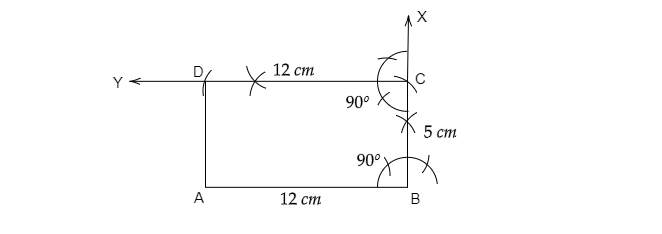# Construct a rectangle $ABCD$, where $AB =12\ cm,\ BC=5\ cm$.

Given: A rectangle $ABCD$, where $AB =12\ cm,\ BC=5\ cm$.

To do: To construct $ABCD$.

Solution:

1. Draw $AB=12\ cm$.

2. At point $B$, draw an angle  $ABX=90^o$.

3. Take point $B$ as center and draw an arc with radius $5\ cm$, which intersects $BX$ at point $C$.

4. At point $C$, draw an angle $YCB=90^o$.

5. Take point $C$ as center, draw an arc with radius $12\ cm$ which intersects $YC$ at point $D$.

6. Join $D$ to $C$.Thus, $ABCD$ is the required rectangle.

Updated on: 10-Oct-2022

38 Views